Refraction and the Ray Model of Light - Lesson 5 - Image Formation by Lenses

# Diverging Lenses - Ray Diagrams

Earlier in Lesson 5, we learned how light is refracted by double concave lens in a manner that a virtual image is formed. We also learned about three simple rules of refraction for double concave lenses:
• Any incident ray traveling parallel to the principal axis of a diverging lens will refract through the lens and travel in line with the focal point (i.e., in a direction such that its extension will pass through the focal point).
• Any incident ray traveling towards the focal point on the way to the lens will refract through the lens and travel parallel to the principal axis.
• An incident ray that passes through the center of the lens will in effect continue in the same direction that it had when it entered the lens.

These three rules will be used to construct ray diagrams. A ray diagram is a tool used to determine the location, size, orientation, and type of image formed by a lens. Ray diagrams for double convex lenses were drawn in a previous part of Lesson 5. In this lesson, we will see a similar method for constructing ray diagrams for double concave lenses.

### Step-by-Step Method for Drawing Ray Diagrams

The method of drawing ray diagrams for a double concave lens is described below.

1. Pick a point on the top of the object and draw three incident rays traveling towards the lens.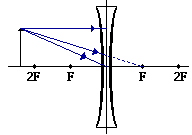Using a straight edge, accurately draw one ray so that it travels towards the focal point on the opposite side of the lens; this ray will strike the lens before reaching the focal point; stop the ray at the point of incidence with the lens. Draw the second ray such that it travels exactly parallel to the principal axis. Draw the third ray to the exact center of the lens. Place arrowheads upon the rays to indicate their direction of travel.

2. Once these incident rays strike the lens, refract them according to the three rules of refraction for double concave lenses.

The ray that travels towards the focal point will refract through the lens and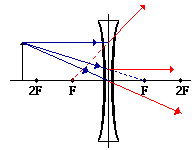travel parallel to the principal axis. Use a straight edge to accurately draw its path. The ray that traveled parallel to the principal axis on the way to the lens will refract and travel in a direction such that its extension passes through the focal point on the object's side of the lens. Align a straight edge with the point of incidence and the focal point, and draw the second refracted ray. The ray that traveled to the exact center of the lens will continue to travel in the same direction. Place arrowheads upon the rays to indicate their direction of travel. The three rays should be diverging upon refraction.

3. Locate and mark the image of the top of the object.

The image point of the top of the object is the point where the three refracted rays intersect.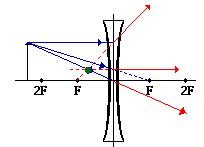Since the three refracted rays are diverging, they must be extended behind the lens in order to intersect. Using a straight edge, extend each of the rays using dashed lines. Draw the extensions until they intersect. All three extensions should intersect at the same location. The point of intersection is the image point of the top of the object. The three refracted rays would appear to diverge from this point. This is merely the point where all light from the top of the object would appear to diverge from after refracting through the double concave lens. Of course, the rest of the object has an image as well and it can be found by applying the same three steps to another chosen point. See note below.

4. Repeat the process for the bottom of the object.

The goal of a ray diagram is to determine the location, size, orientation, and type of image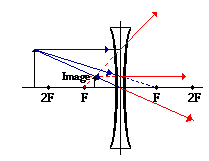that is formed by the double concave lens. Typically, this requires determining where the image of the upper and lower extreme of the object is located and then tracing the entire image. After completing the first three steps, only the image location of the top extreme of the object has been found. Thus, the process must be repeated for the point on the bottom of the object. If the bottom of the object lies upon the principal axis (as it does in this example), then the image of this point will also lie upon the principal axis and be the same distance from the lens as the image of the top of the object. At this point the complete image can be filled in.

Some students have difficulty understanding how the entire image of an object can be deduced once a single point on the image has been determined. If the object is merely a vertical object (such as the arrow object used in the example below), then the process is easy. The image is merely a vertical line. This is illustrated in the diagram below. In theory, it would be necessary to pick each point on the object and draw a separate ray diagram to determine the location of the image of that point. That would require a lot of ray diagrams as illustrated in the diagram below.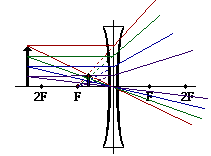Fortunately, a shortcut exists. If the object is a vertical line, then the image is also a vertical line. For our purposes, we will only deal with the simpler situations in which the object is a vertical line that has its bottom located upon the principal axis. For such simplified situations, the image is a vertical line with the lower extremity located upon the principal axis.

The ray diagram above illustrates that the image of an object in front of a double concave lens will be located at a position behind the double concave lens. Furthermore, the image will be upright, reduced in size (smaller than the object), and virtual. This is the type of information that we wish to obtain from a ray diagram. The characteristics of this image will be discussed in more detail in the next section of Lesson 5.

Once the method of drawing ray diagrams is practiced a couple of times, it becomes as natural as breathing. Each diagram yields specific information about the image. It is suggested that you take a few moments to practice a few ray diagrams on your own and to describe the characteristics of the resulting image. The diagrams below provide the setup; you must merely draw the rays and identify the image. If necessary, refer to the method described above.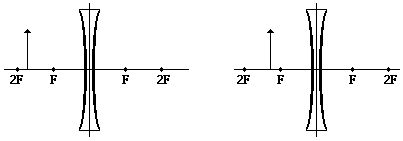### We Would Like to Suggest ...Why just read about it and when you could be interacting with it? Interact - that's exactly what you do when you use one of The Physics Classroom's Interactives. We would like to suggest that you combine the reading of this page with the use of our Optics Bench Interactive. You can find this in the Physics Interactives section of our website. The Optics Bench Interactive provides the learner an interactive enivronment for exploring the formation of images by lenses and mirrors. Its like having a complete optics toolkit on your screen.

Next Section: